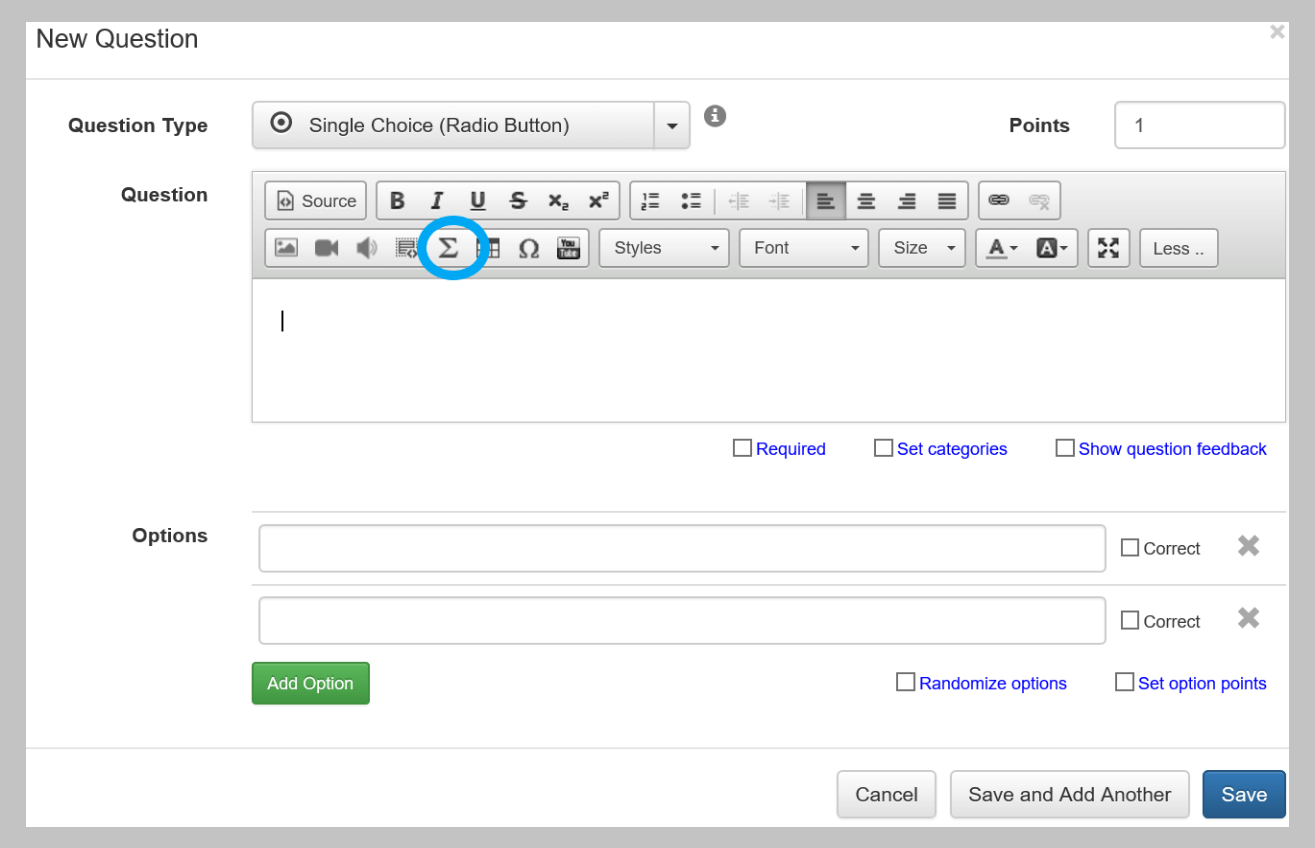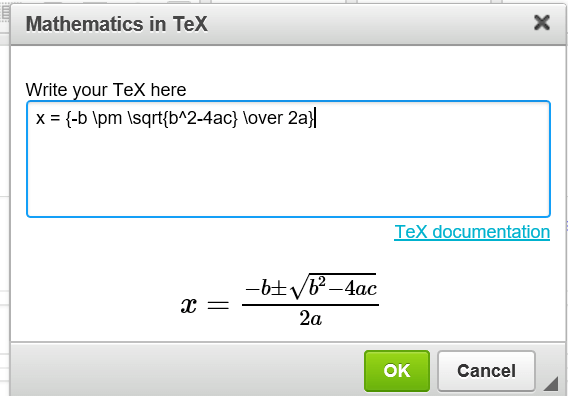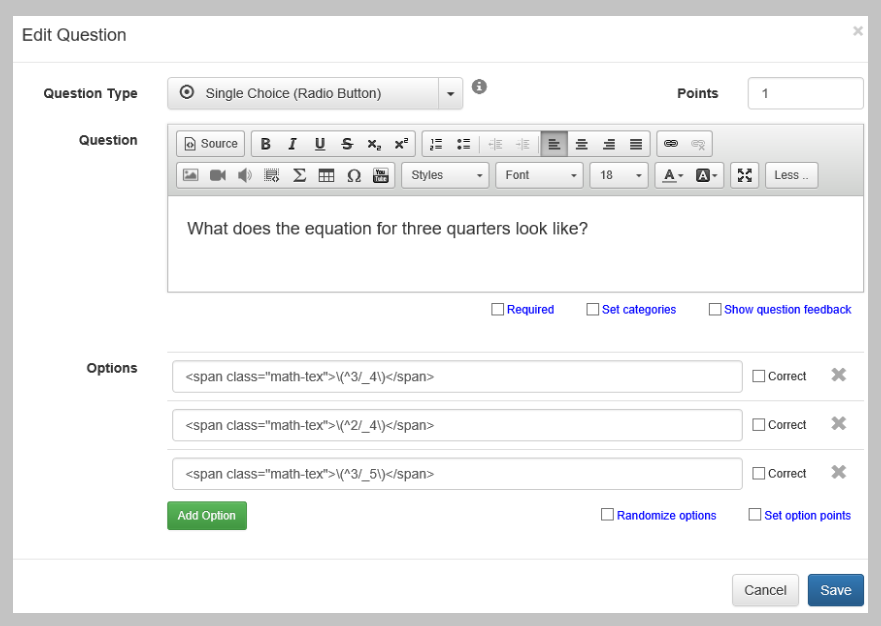Home | Help Guides | How to Make a Quiz with Mathematical Equations

With FlexiQuiz you can create professional looking maths and science tests using complex equations.  This is a step by step guide on how to use TeX Syntax to construct mathematical formulae for including in your quiz, test or assessment.

How to add Equations to a Question

• From the options select more and this displays extra choices
• Select the math button $$\Sigma$$ (highlighted below)• A new window will open
• Enter the relevant TeX syntax and the fraction will be displayed
• For example:  type  ^2/_3  and it will be shown as   $$^2/_3$$  in your question
• Once you have entered the required text press OK and your equation will be saved within your questionYou can also add equations to your Welcome and Thank You pages by following the same process.

How to add an Equation to a Question Option

To insert fractions into Multiple or Single Choice Options, follow these steps.

• Create a question
• In the options box enter the required TeX surrounded by span with the class set to math-tex:
• For example: <span class="math-tex">$$^3/_4$$</span>
• When saved the option will be displayed correctly such as $$^3/_4$$Any Suggestions, Questions or Feedback?  Please let us know!